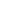# Free Class 7 Formation of algebraic expressions Worksheets

Download free printable Formation of algebraic expressions Worksheets to practice. With thousands of questions available, you can generate as many Formation of algebraic expressions Worksheets as you want.

## Sample Class 7 Formation of algebraic expressions Worksheet Questions

1.

Fill in the blanks: The value of 1 - 5x2 at x - 3 is _______.

2.

Fill in the blanks: The coefficient of y2 in -5xy2 is ________.

3.

Identify the monomials, binomials, trinomials and quadrinomials from the following expressions: 3x2 + 4y2 + 52

4.

In the following expressions write the numerical coefficient of each term: -5/3x2y + 7/4xy2 + 3

5.

In the following expressions write the numerical coefficient of each term: 4x2y - 3/2xy + 5/2xy2

6.

In the following expressions write the numerical coefficient of each term: -2x3y2

7.

In the following expressions write the numerical coefficient of each term: 6abc

8.

In the following expressions write the numerical coefficient of each term: -5y2

9.

In the following expressions write the numerical coefficient of each term: xy

10.

In the following expressions write the numerical coefficient of each term: 3xy + 4

Worksheets by UrbanPro

Our worksheets are designed to help students explore various topics, practice skills and enrich their subject knowledge, to improve their academic performance. Designed by Experts who have extensive experience and expertise in teaching a subject, these worksheets will improve your child's problem-solving skills and subject knowledge in a fun and interactive manner.
Check out our free customized worksheets across school boards, grades, subjects and levels of subject knowledge. You can download, print and share these worksheets with anyone, anywhere, anytime!

Get a custom worksheet to practice!

Select your topic & see the magic.

subjectSelect Chapter(s)

Chapters & Subtopics